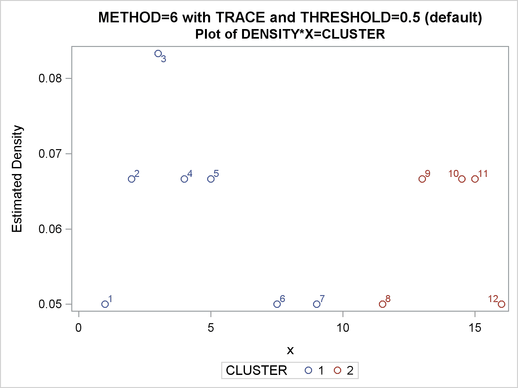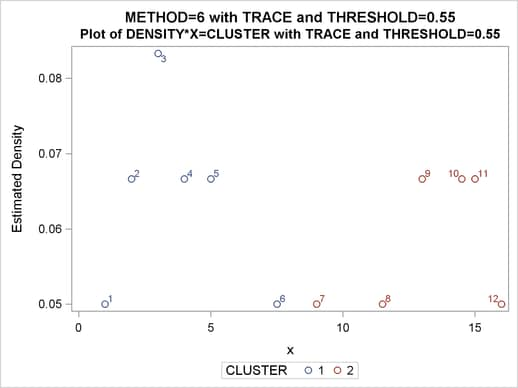## Example 59.5 Using the TRACE Option When METHOD=6

To illustrate how the TRACE option can help you to understand the clustering process when METHOD=6 is specified, the following data set is created with 12 observations:

```data test;
input x @@;
datalines;
1 2 3 4 5 7.5 9 11.5 13 14.5 15 16
;
```

The first five observations seem to be close to each other, and the last five observations seem to be close to each other. Observation 6 is separated from the first five observations with a (Euclidean) distance of 2.5, and the same distance separates observation 7 from the last five observations. Observations 6 and 7 differ by 1.5.

Suppose METHOD=6 with a radius of 2.5 is chosen for the cluster analysis. You can specify the TRACE option to understand how each observation is assigned.

The following statements produce Output 59.5.1 and Output 59.5.2:

```/*-- METHOD=6 with TRACE and THRESHOLD=0.5 (default) --*/
title 'METHOD=6 with TRACE and THRESHOLD=0.5 (default)';

proc modeclus data=test method=6 r=2.5 trace short out=out;
var x;
run;

title2 'Plot of DENSITY*X=CLUSTER';

proc sgplot data=out;
scatter y=density x=x / group=cluster datalabel=_obs_;
run;

```

Output 59.5.1 Partial Output of METHOD=6 with TRACE and Default THRESHOLD=
 METHOD=6 with TRACE and THRESHOLD=0.5 (default)

The MODECLUS Procedure
R=2.5 METHOD=6

Trace of Clustering Algorithm
Obs Density Cluster Ratio
Old New
3 0.0833333 -1 1 M
2 0.0666667 0 1 N
4 0.0666667 0 1 N
5 0.0666667 0 1 N
1 0.0500000 0 1 N
6 0.0500000 0 1 0.571
7 0.0500000 -1 1 0.500
9 0.0666667 -1 2 M
8 0.0500000 0 2 N
10 0.0666667 -1 2 S
12 0.0500000 0 2 N
11 0.0666667 -1 2 S

 METHOD=6 with TRACE and THRESHOLD=0.5 (default)

The MODECLUS Procedure

Cluster Summary
R Number of
Clusters
Frequency of
Unclassified
Objects
2.5 2 0

Output 59.5.2 Density PlotNote that in Output 59.5.1, observation 7 is originally a seed (indicated by a value of –1 in the "Old" column) and then assigned to cluster 1. This is because the ratio of observation 7 to cluster 1 is 0.5 and is not less than the default value of the THRESHOLD= option (0.5).

If the value of the THRESHOLD= option is increased to 0.55, observation 7 should be excluded from cluster 1 and the cluster membership of observation 7 is changed.

The following statements produce Output 59.5.3 and Output 59.5.4:

```/*-- METHOD=6 with TRACE and THRESHOLD=0.55 --*/
title 'METHOD=6 with TRACE and THRESHOLD=0.55';

proc modeclus data=test method=6 r=2.5 trace threshold=0.55 short out=out;
var x;
run;

title2 'Plot of DENSITY*X=CLUSTER with TRACE and THRESHOLD=0.55';

proc sgplot data=out;
scatter y=density x=x / group=cluster datalabel=_obs_;
run;
```

Output 59.5.3 Partial Output of METHOD=6 with TRACE and THRESHOLD=.55
 METHOD=6 with TRACE and THRESHOLD=0.55

The MODECLUS Procedure
R=2.5 METHOD=6

Trace of Clustering Algorithm
Obs Density Cluster Ratio
Old New
3 0.0833333 -1 1 M
2 0.0666667 0 1 N
4 0.0666667 0 1 N
5 0.0666667 0 1 N
1 0.0500000 0 1 N
6 0.0500000 0 1 0.571
9 0.0666667 -1 2 M
8 0.0500000 0 2 N
10 0.0666667 -1 2 S
12 0.0500000 0 2 N
11 0.0666667 -1 2 S
7 0.0500000 -1 2 S

 METHOD=6 with TRACE and THRESHOLD=0.55

The MODECLUS Procedure

Cluster Summary
R Number of
Clusters
Frequency of
Unclassified
Objects
2.5 2 0

Output 59.5.4 Density PlotIn Output 59.5.3, observation 7 is a seed that is excluded by cluster 1 because its ratio to cluster 1 is less than 0.55. Being a neighbor of a member (observation 8) of cluster 2, observation 7 eventually joins cluster 2 even though it remains a "SEED." (See Step 2.2 in the section METHOD=6.)